# Discrete Mathematics and Combinatorics Commons™

1,115 Full-Text Articles 1,295 Authors 292,068 Downloads114 Institutions

## All Articles in Discrete Mathematics and Combinatorics

1,115 full-text articles. Page 3 of 44.

2022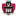University of Arkansas, Fayetteville

#### 3-Uniform 4-Path Decompositions Of Complete 3-Uniform Hypergraphs, Rachel Mccann

##### Mathematical Sciences Undergraduate Honors Theses

The complete 3-uniform hypergraph of order v is denoted as Kv and consists of vertex set V with size v and edge set E, containing all 3-element subsets of V. We consider a 3-uniform hypergraph P7, a path with vertex set {v1, v2, v3, v4, v5, v6, v7} and edge set {{v1, v2, v3}, {v2, v3, v4}, {v4, v5, v6}, {v5, v6 …

Studying Extended Sets From Young Tableaux, 2022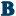Butler University

#### Studying Extended Sets From Young Tableaux, Eric S. Nofziger

Young tableaux are combinatorial objects related to the partitions of an integer that have various applications in representation theory. These tableaux are defined as a left-justified set of n boxes filled with the numbers 1 through n and organized in rows, with the length of each row corresponding to a summand in the partition. In recent work of Graham–Precup–Russell, an association has been made between a given row-strict tableau and three disjoint subsets I, J, and K, also called extended sets. In this project, we begin to classify which extended sets correlate to a valid row-strict or standard tableau. We …

Diederich-Fornæss Index On Boundaries Containing Crescents, 2022University of Arkansas, Fayetteville

#### Diederich-Fornæss Index On Boundaries Containing Crescents, Jason Demoulpied

The worm domain developed by Diederich and Fornæss is a classic example of a boundedpseudoconvex domains that fails to satisfy global regularity of the Bergman Projection, due to the set of weakly pseudoconvex points that form an annulus in its boundary. We instead examine a bounded pseudoconvex domain Ω ⊂ C2 whose set of weakly pseudoconvex points form a crescent in its boundary. In 2019, Harrington had shown that these types of domains satisfy global regularity of the Bergman Projection based on the existence of good vector fields. In this thesis we study the Regularized Diederich-Fornæss index of these domains, …

Modern Theory Of Copositive Matrices, 2022William & Mary

#### Modern Theory Of Copositive Matrices, Yuqiao Li

Copositivity is a generalization of positive semidefiniteness. It has applications in theoretical economics, operations research, and statistics. An $n$-by-$n$ real, symmetric matrix $A$ is copositive (CoP) if $x^T Ax \ge 0$ for any nonnegative vector $x \ge 0.$ The set of all CoP matrices forms a convex cone. A CoP matrix is ordinary if it can be written as the sum of a positive semidefinite (PSD) matrix and a symmetric nonnegative (sN) matrix. When $n < 5,$ all CoP matrices are ordinary. However, recognizing whether a given CoP matrix is ordinary and determining an ordinary decomposition (PSD + sN) is still an unsolved problem. Here, we give an overview on modern theory of CoP matrices, talk about our progress on the ordinary recognition and decomposition problem, and emphasis the graph theory aspect of ordinary CoP matrices.

Quantum Dimension Polynomials: A Networked-Numbers Game Approach, 2022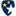Murray State University

#### Quantum Dimension Polynomials: A Networked-Numbers Game Approach, Nicholas Gaubatz

##### Honors College Theses

The Networked-Numbers Game--a mathematical "game'' played on a simple graph--is incredibly accessible and yet surprisingly rich in content. The Game is known to contain deep connections to the finite-dimensional simple Lie algebras over the complex numbers. On the other hand, Quantum Dimension Polynomials (QDPs)--enumerative expressions traditionally understood through root systems--corresponding to the above Lie algebras are complicated to derive and often inaccessible to undergraduates. In this thesis, the Networked-Numbers Game is defined and some known properties are presented. Next, the significance of the QDPs as a method to count combinatorially interesting structures is relayed. Ultimately, a novel closed-form expression of …

How To Guard An Art Gallery: A Simple Mathematical Problem, 2022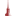St. John Fisher University

#### How To Guard An Art Gallery: A Simple Mathematical Problem, Natalie Petruzelli

##### The Review: A Journal of Undergraduate Student Research

The art gallery problem is a geometry question that seeks to find the minimum number of guards necessary to guard an art gallery based on the qualities of the museum’s shape, specifically the number of walls. Solved by Václav Chvátal in 1975, the resulting Art Gallery Theorem dictates that ⌊n/3⌋ guards are always sufficient and sometimes necessary to guard an art gallery with n walls. This theorem, along with the argument that proves it, are accessible and interesting results even to one with little to no mathematical knowledge, introducing readers to common concepts in both geometry and graph …

2022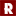Prospect High School, Saratoga

#### A New Method To Compute The Hadamard Product Of Two Rational Functions, Ishan Kar

The Hadamard product (denoted by∗) of two power series A(x) =a0+a1x+a2x2+···and B(x) =b0+b1x+b2x2+··· is the power series A(x)∗B(x) =a0b0+a1b1x+a2b2x2+···. Although it is well known that the Hadamard product of two rational functions is also rational, a closed form expression of the Hadamard product of rational functions has not been found. Since any rational power series can be expanded by partial fractions as a polynomial plus a sum of power series …

2022Bethel University

#### Some Np-Complete Edge Packing And Partitioning Problems In Planar Graphs, Jed Yang

##### Communications on Number Theory and Combinatorial Theory

Graph packing and partitioning problems have been studied in many contexts, including from the algorithmic complexity perspective. Consider the packing problem of determining whether a graph contains a spanning tree and a cycle that do not share edges. Bernáth and Király proved that this decision problem is NP-complete and asked if the same result holds when restricting to planar graphs. Similarly, they showed that the packing problem with a spanning tree and a path between two distinguished vertices is NP-complete. They also established the NP-completeness of the partitioning problem of determining whether the edge set of a graph can be …

2022Louisiana State University and Agricultural and Mechanical College

#### Characterizations Of Certain Classes Of Graphs And Matroids, Jagdeep Singh

##### LSU Doctoral Dissertations

If a theorem about graphs can be expressed in terms of edges and cycles only, it probably exemplifies a more general theorem about matroids." Most of my work draws inspiration from this assertion, made by Tutte in 1979.

In 2004, Ehrenfeucht, Harju and Rozenberg proved that all graphs can be constructed from complete graphs via a sequence of the operations of complementation, switching edges and non-edges at a vertex, and local complementation. In Chapter 2, we consider the binary matroid analogue of each of these graph operations. We prove that the analogue of the result of Ehrenfeucht et. al. does …

Unavoidable Structures In Large And Infinite Graphs, 2022Louisiana State University and Agricultural and Mechanical College

#### Unavoidable Structures In Large And Infinite Graphs, Sarah Allred

##### LSU Doctoral Dissertations

In this work, we present results on the unavoidable structures in large connected and large 2-connected graphs. For the relation of induced subgraphs, Ramsey proved that for every positive integer r, every sufficiently large graph contains as an induced subgraph either Kr or Kr. It is well known that, for every positive integer r, every sufficiently large connected graph contains an induced subgraph isomorphic to one of Kr, K1,r, and Pr. We prove an analogous result for 2-connected graphs. Similarly, for infinite graphs, every infinite connected graph contains an induced subgraph …

The Enumeration Of Minimum Path Covers Of Trees, 2022William & Mary

#### The Enumeration Of Minimum Path Covers Of Trees, Merielyn Sher

A path cover of a tree T is a collection of induced paths of T that are vertex disjoint and cover all the vertices of T. A minimum path cover (MPC) of T is a path cover with the minimum possible number of paths, and that minimum number is called the path cover number of T. A tree can have just one or several MPC's. Prior results have established equality between the path cover number of a tree T and the largest possible multiplicity of an eigenvalue that can occur in a symmetric matrix whose graph is that tree. We …

Prime Labelings On Planar Grid Graphs, 2022University of Pittsburgh - Johnstown

#### Prime Labelings On Planar Grid Graphs, Stephen James Curran

##### Theory and Applications of Graphs

It is known that for any prime p and any integer n such that 1≤np there exists a prime labeling on the pxn planar grid graph PpxPn. We show that PpxPn has a prime labeling for any odd prime p and any integer n such that that p<n≤p2.

Characterizing Edge Betweenness-Uniform Graphs, 2022University of Economics, Tajovského

#### Characterizing Edge Betweenness-Uniform Graphs, Jana Coroničová Hurajová, Tomas Madaras, Darren A. Narayan

##### Theory and Applications of Graphs

The \emph{betweenness centality} of an edge $e$ is, summed over all $u,v\in V(G)$, the ratio of the number of shortest $u,v$-paths in $G$ containing $e$ to the number of shortest $u,v$-paths in $G$. Graphs whose vertices all have the same edge betweenness centrality are called \emph{edge betweeness-uniform}. It was recently shown by Madaras, Hurajová, Newman, Miranda, Fl\'{o}rez, and Narayan that of the over 11.7 million graphs with ten vertices or fewer, only four graphs are edge betweenness-uniform but not edge-transitive.In this paper we present new results involving properties of betweenness-uniform graphs.

Chromatic Polynomials Of Signed Book Graphs, 2022Indian Institute of Technology Guwahati

#### Chromatic Polynomials Of Signed Book Graphs, Deepak Sehrawat, Bikash Bhattacharjya

##### Theory and Applications of Graphs

For $m \geq 3$ and $n \geq 1$, the $m$-cycle \textit{book graph} $B(m,n)$ consists of $n$ copies of the cycle $C_m$ with one common edge. In this paper, we prove that (a) the number of switching non-isomorphic signed $B(m,n)$ is $n+1$, and (b) the chromatic number of a signed $B(m,n)$ is either 2 or 3. We also obtain explicit formulas for the chromatic polynomials and the zero-free chromatic polynomials of switching non-isomorphic signed book graphs.

Moving Polygon Methods For Incompressible Fluid Dynamics, 2022University of Massachusetts Amherst

#### Moving Polygon Methods For Incompressible Fluid Dynamics, Chris Chartrand

##### Doctoral Dissertations

Hybrid particle-mesh numerical approaches are proposed to solve incompressible fluid flows. The methods discussed in this work consist of a collection of particles each wrapped in their own polygon mesh cell, which then move through the domain as the flow evolves. Variables such as pressure, velocity, mass, and momentum are located either on the mesh or on the particles themselves, depending on the specific algorithm described, and each will be shown to have its own advantages and disadvantages. This work explores what is required to obtain local conservation of mass, momentum, and convergence for the velocity and pressure in a …

Minimality Of Integer Bar Visibility Graphs, 2022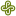Portland State University

#### Minimality Of Integer Bar Visibility Graphs, Emily Dehoff

##### University Honors Theses

A visibility representation is an association between the set of vertices in a graph and a set of objects in the plane such that two objects have an unobstructed, positive-width line of sight between them if and only if their two associated vertices are adjacent. In this paper, we focus on integer bar visibility graphs (IBVGs), which use horizontal line segments with integer endpoints to represent the vertices of a given graph. We present results on the exact widths of IBVGs of paths, cycles, and stars, and lower bounds on trees and general graphs. In our main results, we find …

Application Of The Combinatorial Nullstellensatz To Integer-Magic Graph Labelings, 2022San Jose State University

#### Application Of The Combinatorial Nullstellensatz To Integer-Magic Graph Labelings, Richard M. Low, Dan Roberts

##### Theory and Applications of Graphs

Let $A$ be a nontrivial abelian group and $A^* = A \setminus \{0\}$. A graph is $A$-magic if there exists an edge labeling $f$ using elements of $A^*$ which induces a constant vertex labeling of the graph. Such a labeling $f$ is called an $A$-magic labeling and the constant value of the induced vertex labeling is called an $A$-magic value. In this paper, we use the Combinatorial Nullstellensatz to show the existence of $\mathbb{Z}_p$-magic labelings (prime $p \geq 3$ ) for various graphs, without having to construct the $\mathbb{Z}_p$-magic labelings. Through many examples, we illustrate the usefulness (and limitations) in …

Connectedness Of Unit Distance Subgraphs Induced By Closed Convex Sets, 2022Delft University of Technology

#### Connectedness Of Unit Distance Subgraphs Induced By Closed Convex Sets, Remie Janssen, Leonie Van Steijn

##### Theory and Applications of Graphs

The unit distance graph $G^1_{R^d}$ is the infinite graph whose nodes are points in $R^d$, with an edge between two points if the Euclidean distance between these points is $1$. The 2-dimensional version $G^1_{R^2}$ of this graph is typically studied for its chromatic number, as in the Hadwiger-Nelson problem. However, other properties of unit distance graphs are rarely studied. Here, we consider the restriction of $G^1_{R^d}$ to closed convex subsets $X$ of $R^d$. We show that the graph $G^1_{R^d}[X]$ is connected precisely when the radius of $r(X)$ of $X$ is equal to $0$, or when $r(X)\geq 1$ and the affine …

Facial Achromatic Number Of Triangulations With Given Guarding Number, 2022Keio University

#### Facial Achromatic Number Of Triangulations With Given Guarding Number, Naoki Matsumoto, Yumiko Ohno

##### Theory and Applications of Graphs

A (not necessarily proper) $k$-coloring $c : V(G) \rightarrow \{1,2,\dots,k\}$ of a graph $G$ on a surface is a {\em facial $t$-complete $k$-coloring} if every $t$-tuple of colors appears on the boundary of some face of $G$. The maximum number $k$ such that $G$ has a facial $t$-complete $k$-coloring is called a {\em facial $t$-achromatic number} of $G$, denoted by $\psi_t(G)$. In this paper, we investigate the relation between the facial 3-achromatic number and guarding number of triangulations on a surface, where a {\em guarding number} of a graph $G$ embedded on a surface, denoted by $\gd(G)$, is the smallest …

Generating B-Nomial Numbers, 2022Shippensburg University of Pennsylvania

#### Generating B-Nomial Numbers, Ji Young Choi

##### Communications on Number Theory and Combinatorial Theory

This paper presents three new ways to generate each type of b-nomial numbers: We develop ordinary generating functions, we find a whole new set of recurrence relations, and we identify each b-nomial number as a single binomial coefficient or as an alternating sum of products of two binomial coefficients.